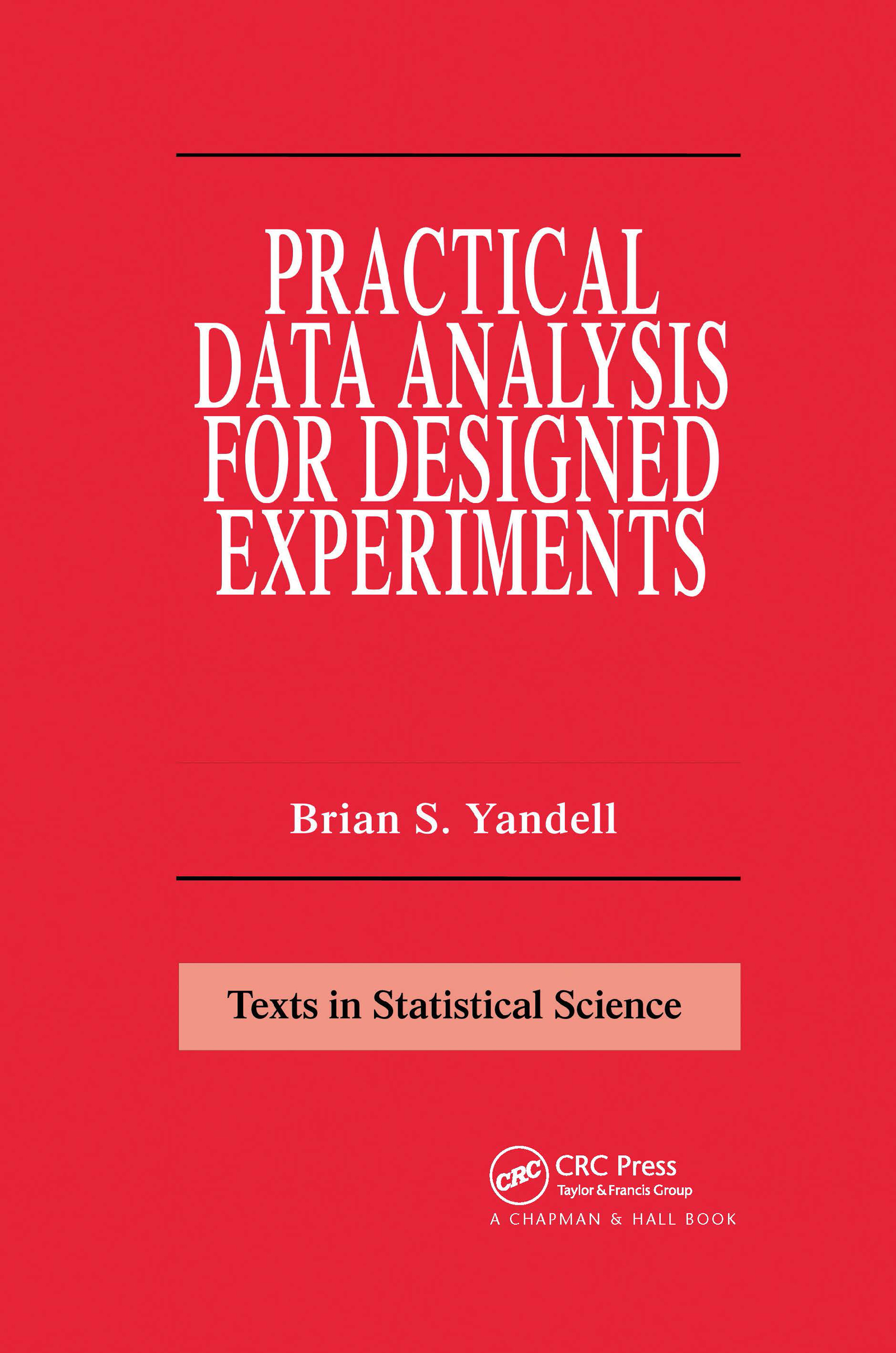Practical Data Analysis for Designed Experiments

1st Edition

Chapman and Hall/CRC

440 pages

Purchasing Options:\$ = USD
Hardback: 9780412063411
pub: 1997-01-01
SAVE ~\$31.00
\$155.00
\$124.00
x
eBook (VitalSource) : 9780203742563
pub: 2017-11-22
from \$28.98

FREE Standard Shipping!

Description

Placing data in the context of the scientific discovery of knowledge through experimentation, Practical Data Analysis for Designed Experiments examines issues of comparing groups and sorting out factor effects and the consequences of imbalance and nesting, then works through more practical applications of the theory. Written in a modern and accessible manner, this book is a useful blend of theory and methods. Exercises included in the text are based on real experiments and real data.

Reviews

"…the book should be useful for statisticians who are starting out as consultants…also contains much good practical advice based on the writer's experience as a teacher and statistical advisor."

-M. Talbot, Biometrics, December 1998

"…gives a generally lucid and well thought out introduction to the use of data driven approaches for statistical data analysis…the explanations are clear, without being obscured by too much mathematical detail…an excellent basis for a statistics course with an applied orientation, and most institutions that teach statistics or analyse data will probably want a library copy."

-S.N. Wood,Biometrics,December 1998

Preface

Part A: Placing Data in Context

Practical Data Analysis

Effect of Factors

Nature of Data

Summary Tables

Plots for Statistics

Computing

Interpretation

Problems

Collaboration in Science

Learning from Plots

Mechanics of a Consulting Session

Philosophy and Ethics

Intelligence, Culture and Learning

Writing

Problems

Experimental Design

Types of Studies

Designed Experiments

Design Structure

Treatment Structure

Designs in This Book

Problems

Part B: Working with Groups of Data

Group Summaries

Graphical Summaries

Estimates of Means and Variance

Assumptions and Pivot Statistics

Interval Estimates of Means

Testing Hypotheses about Means

Formal Inference on the Variance

Problems

Comparing Several Means

Linear Contrasts of Means

Overall Test of Difference

Partitioning Sums of Squares

Expected Mean Squares

Power and Sample Size

Problems

Multiple Comparisons of Means

Experiment- and Comparison-Wise Error Rates

Comparisons Based on F-Tests

Comparisons Based on Range of Means

Comparisons of Comparisons

Problems

Part C: Sorting Out Effects with Data

Factorial Designs

Cell Means Models

Effects Models

Estimable Functions

Linear Constraints

General Form of Estimable Functions

Problems

Balanced Experiments

Full Models with Two Factors

Interaction Plots

Higher Orders Models

Problems

Model Selection

Pooling Interactions

Selected the "Best" Model

Model Selection Criteria

One Observation per Cell

Tukey's Test for Interaction

Problems

Part D: Dealing with Imbalance

Unbalanced Experiments

Unequal Samples

Types I, II, III and IV

Problems

Missing Cells

What Are Missing Cells?

Connected Cells and Incomplete Designs

Type IV Comparisons

Latin Square Designs

Fractional Factorial Designs

Problems

Linear Models Inference

Matrix Preliminaries

Ordinary Least Squares

Weighted Least Squares

Maximum Likelihood

Restricted Maximum Likelihood

Inference for Fixed Effect Models

Anova and Regression Models

Problems

Part E: Questioning Assumptions

Residual Plots

Departures from Assumptions

Incorrect Model

Correlated Responses

Unequal Variance

Non-Normal Data

Problems

Comparisons with Unequal Variance

Comparing Means When Variances Are Unequal

Weighted Analysis of Variances

Satterthwaite Approximation

Generalized Inference

Testing for Unequal Variances

Problems

Getting Free from Assumptions

Transforming Data

Comparisons Using Ranks

Randomization

Monte Carlo Methods

Problems

Part F: Regressing with Factors

Ordered Groups

Groups in a Line

Testing for Linearity

Path Analysis Diagrams

Regression Calibration

Classical Error in Variables

Problems

Parallel Lines

Parallel Lines Model

Plots with Symbols

Sequential Tests with Multiple Responses

Sequential Tests with Driving Covariate

Adjusted (Type III) Tests of Hypotheses

Different Slopes for Different Groups

Problems

Multiple Responses

Overall Tests for Group Differences

Matrix Analog to F Test

How Do Groups Differ?

Causal Models

Part G: Deciding on Fixed or Random Effects

Models with Random Effects

Single Factor Random Model

Test for Class Variation

Distribution of Sums of Squares

Variance Components

Problems

General Random Models

Two Factor Random Models

Unbalanced Two-Factor Random Model

General Random Model

Quadratic Forms in Random Effects

Application to Two Factor Random Model

Problems

Mixed Effect Models

Two Factor Mixed Models

General Mixed Models

Problems

Part H: Nesting Experimental Units

Nested Designs

Sub-Sampling

Blocking

Nested and Crossed Factors

Nesting of Fixed Effects

Nesting of Random Effects

Problems

Split Plot Design

Several Views of Split Plot

Split Plot Model

Contrasts in a Split Plot

Problems

General Nested Designs

Extensions of Split Plot

Split Plot

Imbalance in Nested Designs

Covariates in Nested Designs

Explained Variation in Nested Designs

Problems

Part I: Repeating Measures on Subjects

Repeated Measures as Split Plot

Repeated Measures Designs

Repeated Measures Model

Split Plot More or Less

Expected Mean Squares under Sphericity

Contrasts under Sphericity

Problems

Adjustments to Split Plot

Contrasts over Time

Multivariate Repeated Measures

Problems

Cross-Over Design

Cross-Over Model

Confounding in Cross-Over Designs

Partition of Sum of Squares

Replicated Latin Square Design

General Cross-Over Designs

Problems

References

Index